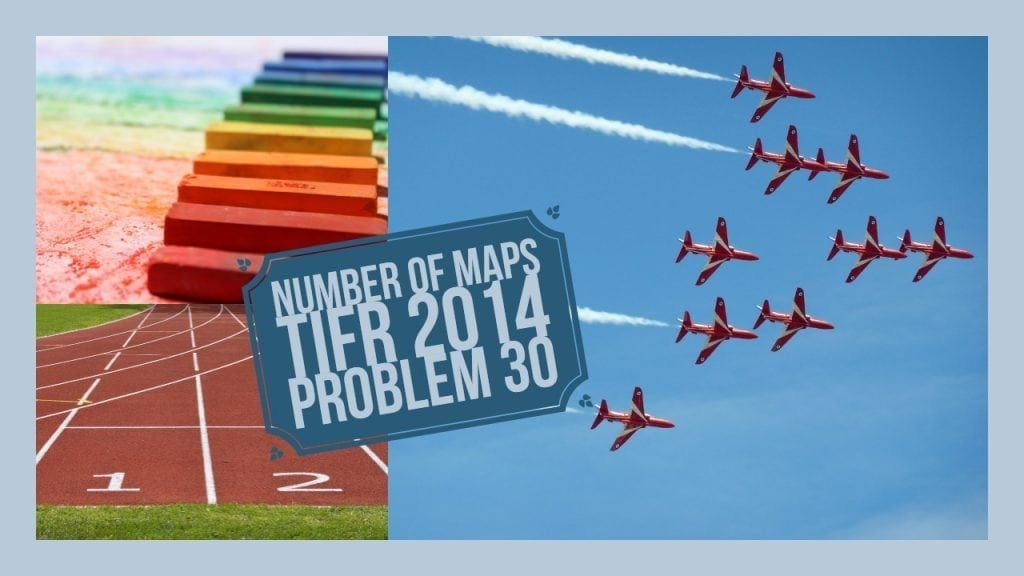How Cheenta works to ensure student success?
Explore the Back-Story

# TIFR 2014 Problem 30 Solution - Number of MapsTIFR 2014 Problem 30 Solution is a part of TIFR entrance preparation series. The Tata Institute of Fundamental Research is India's premier institution for advanced research in Mathematics. The Institute runs a graduate programme leading to the award of Ph.D., Integrated M.Sc.-Ph.D. as well as M.Sc. degree in certain subjects.
The image is a front cover of a book named Topics in Algebra by I.N.Herstein. This book is very useful for the preparation of TIFR Entrance.

Also Visit: College Mathematics Program

## PROBLEM:

How many maps $\phi: \mathbb{N} \cup {0} \to \mathbb{N} \cup {0}$ are there satisfying $\phi(ab)=\phi(a)+\phi(b)$ , for all $a,b\in \mathbb{N} \cup {0}$ ?

## Discussion:

Take $n\in \mathbb{N} \cup {0}$.

By the given equation $\phi(n\times 0)=\phi(n)+\phi(0)$.

This means $\phi(0)=\phi(n)+\phi(0)$.

Oh! This means $\phi(n)=0$. $n\in \mathbb{N} \cup {0}$ was taken arbitrarily. So...

$\phi(n)=0$ for all $n\in \mathbb{N} \cup {0}$.

There is only one such map.

## HELPDESK

• What is this topic:Algebra
• What are some of the associated concept: Number of Function
• Book Suggestions: Topics in Algebra by I.N.HersteinTIFR 2014 Problem 30 Solution is a part of TIFR entrance preparation series. The Tata Institute of Fundamental Research is India's premier institution for advanced research in Mathematics. The Institute runs a graduate programme leading to the award of Ph.D., Integrated M.Sc.-Ph.D. as well as M.Sc. degree in certain subjects.
The image is a front cover of a book named Topics in Algebra by I.N.Herstein. This book is very useful for the preparation of TIFR Entrance.

Also Visit: College Mathematics Program

## PROBLEM:

How many maps $\phi: \mathbb{N} \cup {0} \to \mathbb{N} \cup {0}$ are there satisfying $\phi(ab)=\phi(a)+\phi(b)$ , for all $a,b\in \mathbb{N} \cup {0}$ ?

## Discussion:

Take $n\in \mathbb{N} \cup {0}$.

By the given equation $\phi(n\times 0)=\phi(n)+\phi(0)$.

This means $\phi(0)=\phi(n)+\phi(0)$.

Oh! This means $\phi(n)=0$. $n\in \mathbb{N} \cup {0}$ was taken arbitrarily. So...

$\phi(n)=0$ for all $n\in \mathbb{N} \cup {0}$.

There is only one such map.

## HELPDESK

• What is this topic:Algebra
• What are some of the associated concept: Number of Function
• Book Suggestions: Topics in Algebra by I.N.Herstein

This site uses Akismet to reduce spam. Learn how your comment data is processed.

### Knowledge Partner